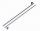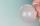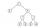# Fence

Square garden has an area of 537 m2. How many meters netting is necessary to fence the garden?

Result

x =  92.693 m

#### Solution:Leave us a comment of example and its solution (i.e. if it is still somewhat unclear...):Be the first to comment!## Next similar examples:

1. Garden plotCalculate how many meters of fence need to fence the square garden with length and width of 22 meters.
2. Perimeter from areaWhat is the perimeter of the square if its content is 64 cm2?
3. Area of squareCalculate the content area of the square whose perimeter is 24 dm.
4. PlaygroundRectangular playground is fenced with 38 m long netting. Its width is 7 m. Calculate its length.
5. AreaCalculate: ?
6. The resultHow many times I decrease the number 1632 to get the result 24?
7. Flood waterFlood waters in some US village meant that the homes had to evacuate 364 people. 50 of them stayed at elementary schools, 59 them slept with their friends and others went to relatives. How many people have gone to relatives?
8. PlaneOn how many parts divide plane 6 parallels?
9. CakesOn the bowl were a few cakes. Jane ate one-third of them, Dana ate a quarter of those cakes that remained. a) What part (of the original number of cakes) Dana ate? b) At least how many cakes could be (initially) on thebowl?
10. Ten pupils10 pupils went to the store. 6 pupils bought lollipops and 9 pupils bought chewing gum. How many pupils have bought both lollipops and chewing gums (if everyone bought something)?
11. SimplifySimplify the following problem and express as a decimal: 5.68-[5-(2.69+5.65-3.89) /0.5]
12. ExpressionSolve for a specified variable: P=a+4b+3c, for aAdded together and write as decimal number: LXVII + MLXIVCalculate perimeter of the rectangle with sides a=2.4 m and b=1.9 m.Find out value of expressions if a = -1, b =2: x=b - 2a - ab y=a3 - b2 - 2ab z=a2 b3 - a3 b2 w=a + b + a3 - b2The hotel has a p floors each floor has i rooms from which the third are single and the others are double. Represents the number of beds in hotel.Solve equation: 4(a-3)=3(2a-5)